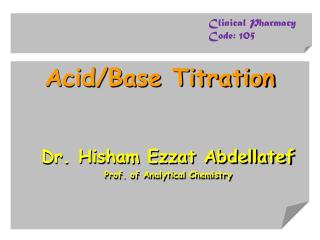DownloadDownload PresentationAcid/Base Titration

# Acid/Base Titration

Télécharger la présentation## Acid/Base Titration

- - - - - - - - - - - - - - - - - - - - - - - - - - - E N D - - - - - - - - - - - - - - - - - - - - - - - - - - -
##### Presentation Transcript

1. Clinical Pharmacy Code: 105 Acid/Base Titration Dr. HishamEzzatAbdellatef Prof. of Analytical Chemistry

2. 1 2 4 3

3. Electrolyte and the theory of electrolytic Dissociation • Electrolytes • conduct the electric current • mineral acid, caustic alkalies and salts • non–electrolytes • non–conducting solutions • cane sugar, glycerin and ethyl acetate.

4. Acid–Base Titration • Pure water is a bad conductor of electricity • acid as HCl H+ Cl- • base as KOH K+ OH- • salt as Na2SO4 Na+ SO42- • Molecule Dissociation ions Dissolved in water

5. Strong and weak electrolytes • NaCl ⇌ Na+ + Cl– • K2SO4 ⇌ 2K+ + SO42– • Na2HPO4 ⇌ 2Na+ + H+ + PO43 • Arrhenius therefore introduced a quantity "a", called "the degree of dissociation“ • "a“ = 1 "strong electrolyte“ • “a” very far from unity.= weak electrolyte

6. Law of mass action • "The rate of a chemical reaction is proportional to the active masses of the reacting substances." diluted solution concentration

7. Law of mass action • Vf = [A].[B]. Kf • Vb = [C]. [D]. Kb • Kf [A]. [B] = Kb [C]. [D] "equilibrium constant"

8. The dissociation of water • H2O ⇌ H+ +  OH– • [H+] . [OH] = Kw………..(2) • "The ionic product of water"

9. The dissociation of water • 25oC; the value of Kw [H+] . [OH] = 1014 [H+]2 = Kw = 1 x 1014 ………(3)

10. Hydrogen ion exponent (pH): [H+] 10–6, 10–51 x 10710–8 , 10–9 Acid base Neutral

11. Procedure for Titration • pH is defined as equal to the logarithm of the hydrogen ion concentration with a negative sign. pH = –log [H+]

12. The pH scale 1 2 3 4 5 678 9 10 11 12 13 14 acid neutral base Stomach juice: pH = 1.0 – 3.0 Human blood: pH = 7.3 – 7.5 Lemon juice: pH = 2.2 – 2.4 Seawater: pH = 7.8 – 8.3 Vinegar: pH = 2.4 – 3.4 Ammonia: pH = 10.5 – 11.5 Carbonated drinks: pH = 2.0 – 4.0 0.1M Na2CO3: pH = 11.7 Orange juice: pH = 3.0 – 4.0 1.0M NaOH: pH = 14.0

13. Figure: A pH meter

14. p pKw = pH + pOH  pH = pKw – pOH or pH = 14 – pOH or pOH = 14 – pH

15. Acids and bases: • Arrhenius theory • Acid [H+] • when dissolved in water • Bases, [OH–]

16. Acid and base

17. pH calculations 1. Solution of strong acids and strong bases [H+] or [OH–] =concentration

18. Example 1 • Calculate the pH value of a solution of a completely ionised 1.0 N solution of acid; or base. ? • Solution: [H+] = 1M pH = –log 1 = 0 (zero) similarly, in a completely ionised 1.0 N solution of base [OH–] = 1 M pOH = –log 1 = 0 (zero)

19. Example 2 Calculate the [H+] and pH of 0.009 N hydrochloric acid? solution [H+] = 0.009 N pH =– log (9.0 X 10–3) = – log 9.0 – log 10–3 pH = – 0.95 + 3.00 = 2.05

20. Example 3 • Calculate the pH values of a solution of sodium hydroxide whose [OH–] is 1.05 x 10–3? solution pOH = – (log 1.05 + log 10–3) pOH = – (0.02 –3 ) = 2.98 pH = 14 – 2.98 = 11.02

21. Example 4 • Calculate the hydrogen ion concentration of a solution of pH 5.3? solution pH = – log [H+] 5.3 = –log [H+] [H+] = 5.01 x 10–6 M

22. Example 5 • Calculate the hydroxyl ion concentration of a solution of pH 10.75 ? solution pOH = 14 – 10.75 = 3.25 [OH–] = the antilog of –3.25 [OH–] = 5.62 x 10–4 M

23. pH calculations • 2. Solution of weak acids and bases • A) Calculation of pH of solution of weak acids pH = ½ (pKa + pCa)

24. B) Calculation of pH of solution of weak bases: pH = pKw – ½ (pKb + pCb)

25. pH of salts • A) Salts of strong acids or bases KCl K+ + Cl- K+ + OH- ⇌ KOH Cl- + H+ ⇌ HCl The equilibrium between the hydrogen and hydroxyl ions in water H2O ⇌ H+ +  OH–

26. B) Salts of weak acids or bases (hydrolysis) • Salts of weak acids (or bases) react with water to give basic (or acidic) solutions • Hydrolysis reverse of neutralization

27. i) Salts of weak acids and strong bases: H2O + CH3COO ⇌ CH3COOH + OH • pH = ½ (pKw – pCa + pKa)

28. ii) Salts of weak bases and strong acids: NH4+ + H2O ⇌ NH4OH + H+ pH = ½ (pKw + pCs – pKb)

29. acidic

30. iii) Salts of weak acids and weak bases: pH = ½ pKw + ½ pKa – ½pKb

31. Buffer solutions • Resist changes in pH caused by addition of small amounts of acid or base; or upon dilution.

32. Types of buffers